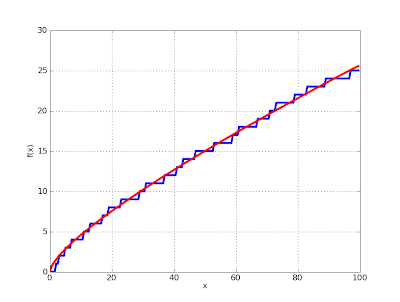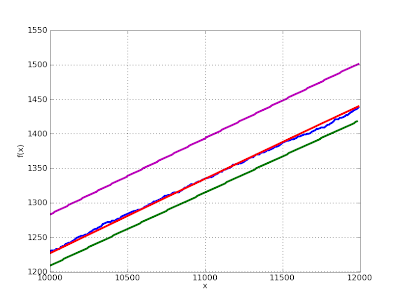# Approximate prime counting

February 17, 2009

I’m continuing my quest for the special functions coverage in mpmath to match the coverage in Mathematica. Comparisons are not quite fair, because mpmath is (with a few exceptions) numerical-only, and it is missing some heavy-duty functions such as the Meijer G-function (and will for the foreseeable future). Many functions in mpmath are also implemented on smaller domains and less rigorously. On the other hand, Mathematica is more restricted in that it only supports machine-precision exponents. And most importantly, the Mathematica source code is very difficult to read for most people.

In any case, I’m trying to catch up with Mathematica 7, which added a whole slew of functions (not that I’ve caught up with Mathematica 6 or even 5). I did add a few functions in version 0.11 of mpmath as a consequence of seeing them in Mathematica 7 (as I recall right now, the Barnes G-function, hyperfactorial and generalized Stieltjes constants). See also the “Fun with zeta functions” post, which discussed the addition of the Riemann-Siegel functions in mpmath.

I just committed an implementation of the Riemann R function R(x) (also discovered in the “new in Mathematica 7″ list), which is an analytic function that closely approximates the prime counting function π(x). The incredible accuracy of the Riemann R function approximation can be visualized by plotting it against the exact prime counting funtion (I added a naive implementation of π(x) as primepi, mainly to facilitate this kind of comparison — SymPy contains a slightly more optimized primepi which could be used instead):

>>> from mpmath import *>>> plot([primepi, riemannr], [0,100])The accuracy for small x is not a fluke, either:

>>> plot([primepi, riemannr], [100000,105000])The “classical” approximation based on the logarithmic integral is not nearly as good:

>>> plot([primepi, lambda x: li(x) - li(2)], [100000,105000])The largest exact value of the prime counting function ever computed was π(1023). The Riemann R function is quite easy to evaluate for x far larger than that, and the relative accuracy only improves:

>>> mp.dps = 50>>> print riemannr(10**1000)4.3448325764011974554109300516481778421781159070188e+996

It is quite likely that no one will ever compute the exact integer that is π(101000), but the value shown above is correct to every indicated digit. In fact, R(101000) can be shown using simple estimates to be accurate to about 500 digits.

For the fun of it, I implemented a version of the prime counting function that quickly returns a strict bounding interval for π(x), using an inequality by Lowell Schoenfeld:This gives for example:

>>> mp.dps = 15>>> print primepi2(10**9)[50823160.0, 50875310.0]

Comparing the Riemann R function and the exact prime counting function with the lower and upper bounds of the Schoenfeld inequality:

>>> pi1 = lambda x: primepi2(x).a>>> pi2 = lambda x: primepi2(x).b>>> plot([primepi, riemannr, pi1, pi2], [10000,12000])Returning to the example x = 101000, the bounds obtained are:

>>> mp.dps = 1000>>> v = primepi2(10**1000)>>> print v.a4344832576401197455410930051648177842178115907018753520641877406789202895629742141091652903064607718165071467289250162191865437538175693583349276176983162304817200288422839930447222611946361773708166613649511865077141255564428528876728476273763948755679190328683931767101119581057633512778001576529635483244120948895852940270929343735968992442702495339548061518980068329967842108151105502256086735702708401375364414161249392284219849396842747077740262581952863984834051417932416644877138920521584769223323611487747350418739878648537734638421782508735661222272164076496151428324862762772509621406939237565543669854423809507199075669125082789947534727993213855318461906160646707017635974631675017156972857845295824952837298472639509394842782539243804497572144916796756352176142163031921644787290530029234804577579707557749789078595077525265053645288083485194663875002648919670801486005071068990914291696975675686030935121708700243252203694578706535251850297014373844901850360974589491752273505884338.0>>> print v.b4344832576401197455410930051648177842178115907018753520641877406789202895629742141091652903064607718165071467289250162191865437538175693583349276176983162304817200288422839930447222611946361773708166613649511865077141255564428528876728476273763948755679190328683931767101119581057633512778001576529635483244120948895852940270929343735968992442702495339548061518980068329967842108151105502256086735702708401375364414161249392284219849396842747077740262581952863984834051417932416644877138920521603092613295597181269170104433360015451521965223364742414453272450659125521224590219319931601764655406966919866611954863306151010025269888571358859446324550862963908106206882475628658096023618959670013112886111645738414968101094745943938600966894156016440525536572293671667091549374215308487923845943703593204613524632469949425914711588697691782286908198073032336714125348156636382686152398749263039694035970181319549445806688213828419222097880911972939154885202053608277109394003350202108994842650400209.0>>> nprint((v.delta)/v.a)4.21728e-495>>> nprint((riemannr(10**1000)-v.a)/v.a)2.10863e-495

and so it turns out that “accurate to about 500 digits” can be replaced by “accurate to at least 494 digits”.

Actually, this is not quite true. Schoenfeld’s inequality depends on the truth of the Riemann hypothesis. Thus, it is conceivable that primepi2 might return an erroneous interval. If you find an output from primepi2 that you can prove to be wrong, this must mean either that 1) you’ve found a bug in mpmath, or 2) you’ve disproved the Riemann hypothesis. (One of these options is more likely than the other, and reports should therefore be submitted to the mpmath issue tracker rather than Annals of Mathematics.)

On a final note, I’ve also added the function zetazero for computing the nth nontrivial zero of the Riemann zeta function:

>>> mp.dps = 50>>> print zetazero(1)(0.5 + 14.134725141734693790457251983562470270784257115699j)>>> print zetazero(2)(0.5 + 21.022039638771554992628479593896902777334340524903j)>>> print zetazero(10)(0.5 + 49.773832477672302181916784678563724057723178299677j)>>> nprint(zeta(zetazero(10)))(2.14411e-50 - 4.15422e-50j)

It just uses a table to determine correct initial values for the rootfinder, so zetazero currently only works up to n = 100. In fact, it works up to n = 100,000 on an internet-enabled computer; zetazero will automatically try to download the table of 100,000 zeros from Andrew Odlyzko’s website if called with n > 100. Or it can load a custom url/file if specified by the user. This is one reason why I love Python: downloading a data file, parsing it and converting it to an appropriate internal representation in virtually a single line of code, easily done in the middle of some algorithmic code.

More information about the Riemann R function can be found in “The encoding of the prime distribution by the zeta zeros“, part of Matthew R. Watkins‘ amazing website. The moral of that document is that the error between the exact prime counting and the Riemann R function can be represented by a kind of “Fourier series” involving a summation over the zeros of the Riemann zeta function. I have not gotten to looking at that yet.